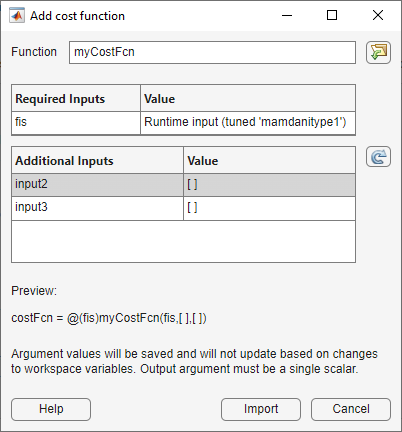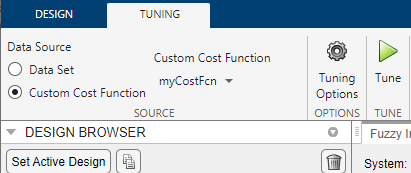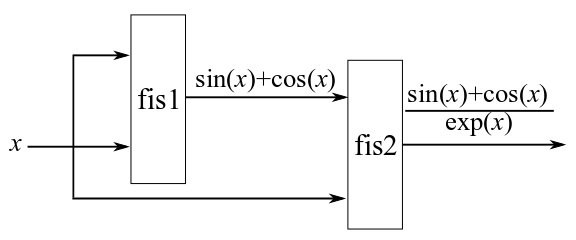## Customize FIS Tuning Process

You can customize your FIS tuning process by either:

For more information on tuning a FIS, see Tuning Fuzzy Inference Systems.

### Specify Custom Cost Function in Fuzzy Logic Designer

To specify a custom cost function when tuning a FIS using Fuzzy Logic Designer, on the Tuning tab, select Custom Cost Function.

Then, in the Custom Cost Function drop-down list, select Add a Custom Cost Fcn.For this example, consider the following cost function, `myCostFcn`, which simulates a Simulink model using the specified FIS.

• The first input of the cost function is the FIS object, which is a required input.

• The other inputs are the name of the Simulink model and the FIS variable name in the MATLAB workspace. Specifying additional inputs is optional.

• The cost function updates the FIS variable in the workspace and simulates the model. It then extracts the reference and actual outputs from the model and computes the root mean square error.

• The output of the cost function is the scalar cost value, which in this case is the root mean square error.

```function cost = myCostFcn(fis,model,fisVarName) % Evaluate model, generate output, and find the cost. % Update workspace variable for the controller. assignin('base',fisVarName,fis) % Get simulation output. out = sim(model); % Get output and reference values from simulation output. outVal = out.yout.signals(1).values; refVal = out.yout.signals(2).values; tout = out.yout.time; % Calculate error from the nominal value. err = outVal - refVal; % Calculate cost as the root mean square of the error. cost = sqrt(mean(err.^2)); end```

In the Add cost function dialog box, in the Function field, enter the name of your custom cost function. When you do so, the Required Inputs table shows the FIS input argument and the Additional Inputs table shows the other inputs.Specify values for the additional inputs by selecting previously defined variables from the MATLAB workspace. For this example:

• `input2` corresponds to the Simulink model name. Select the `modelName` variable.

• `input3` corresponds to the FIS name in model workspace. Select the `fisName` variable.

The values of these variables are saved in the app with the cost function. Therefore, their values do not change when the variable in the MATLAB workspace changes. For this example, if the model name or FIS name change, you must add a new custom cost function in the app.The dialog box shows a preview of the cost function signature. To add this cost function to the app, click .

The app imports and selects the custom cost function.### Tune FIS Using Custom Cost Function

You can use a custom cost function to combine the outputs of the component FISs of a FIS tree using mathematical operations.

As an example, consider the FIS tree from Tune Fuzzy Trees.Suppose you want to modify the FIS tree as shown in the following diagram, combining the FIS outputs using known mathematical operations from the training data.Create the FIS tree, which contains three FIS objects. The outputs of the FIS tree are the outputs of the individual FIS objects.

```fis1 = sugfis('Name','fis1'); fis1 = addInput(fis1,[0 10],'NumMFs',3,'MFType','gaussmf'); fis1 = addOutput(fis1,[-1 1],'NumMFs',3); fis2 = sugfis('Name','fis2'); fis2 = addInput(fis2,[0 10],'NumMFs',3,'MFType','gaussmf'); fis2 = addOutput(fis2,[-1 1],'NumMFs',3); fis3 = sugfis('Name','fis3'); fis3 = addInput(fis3,[0 10],'NumMFs',3,'MFType','gaussmf'); fis3 = addOutput(fis3,[0 1],'NumMFs',3); con = ["fis1/input1" "fis2/input1";"fis2/input1" "fis3/input1"]; fisT = fistree([fis1 fis2 fis3],con);```

Generate training data.

```x = (0:0.1:10)'; y1 = sin(x)+cos(x); y2 = y1./exp(x); y = [y1;y2];```

To implement the addition and multiplication operations, use a cost function. For this example, use the custom function `customcostfcn`, included at the end of the example. Learn a rule base using this cost function.

```options = tunefisOptions('Method',"particleswarm",'OptimizationType',"learning"); options.MethodOptions.MaxIterations = 5; rng('default') fisTout1 = tunefis(fisT,[],@(fis)customcostfcn(fis,x,y),options);```
``` Best Mean Stall Iteration f-count f(x) f(x) Iterations 0 100 0.746 1.31 0 1 200 0.5089 1.249 0 2 300 0.5089 1.086 1 3 400 0.5089 1.112 2 4 500 0.5089 1.106 3 5 600 0.4999 1.051 0 Optimization ended: number of iterations exceeded OPTIONS.MaxIterations. ```

Next, tune all the parameters of the FIS tree.

```options.Method = 'patternsearch'; options.MethodOptions.MaxIterations = 25; [in,out,rule] = getTunableSettings(fisTout1); rng('default') fisTout2 = tunefis(fisTout1,[in;out;rule],@(fis)customcostfcn(fis,x,y),options);```
```Iter Func-count f(x) MeshSize Method 0 1 0.499882 1 1 17 0.499882 2 Successful Poll 2 21 0.499882 1 Refine Mesh 3 64 0.499882 0.5 Refine Mesh 4 128 0.499882 0.25 Refine Mesh 5 143 0.499309 0.5 Successful Poll 6 206 0.499309 0.25 Refine Mesh 7 216 0.497834 0.5 Successful Poll 8 237 0.49493 1 Successful Poll 9 279 0.49493 0.5 Refine Mesh 10 341 0.49493 0.25 Refine Mesh 11 405 0.49493 0.125 Refine Mesh 12 463 0.490647 0.25 Successful Poll 13 527 0.490647 0.125 Refine Mesh 14 567 0.490257 0.25 Successful Poll 15 631 0.490257 0.125 Refine Mesh 16 673 0.486303 0.25 Successful Poll 17 737 0.486303 0.125 Refine Mesh 18 748 0.485744 0.25 Successful Poll 19 812 0.485744 0.125 Refine Mesh 20 884 0.485744 0.0625 Refine Mesh 21 921 0.484482 0.125 Successful Poll 22 932 0.484045 0.25 Successful Poll 23 996 0.484045 0.125 Refine Mesh 24 1067 0.484045 0.0625 Refine Mesh 25 1080 0.483977 0.125 Successful Poll 26 1151 0.483977 0.0625 Refine Mesh Maximum number of iterations exceeded: increase options.MaxIterations. ```

You can add more input/output MFs and specify additional FIS tree outputs to improve the tuning performance. Using additional MF parameters and more training data for additional FIS tree outputs can further fine-tune the outputs of `fis1`, `fis2`, and `fis3`.

Custom Cost Function

```function cost = customcostfcn(fis,x,y) tY = evalfis(fis,x); sincosx = tY(:,1)+tY(:,2); sincosexpx = sincosx.*tY(:,3); actY = [sincosx;sincosexpx]; d = y(:)-actY; cost = sqrt(mean(d.*d)); end```

### Tune FIS Using Custom Optimization Method

You can also implement your own FIS parameter optimization method using `getTunableSettings`, `getTunableValues`, and `setTunableValues`. This example uses these functions to tune a rule base of a fuzzy system.

Create a FIS to approximate $\mathrm{sin}\left(\theta \right)$, where $\theta$ varies from 0 to $2\pi$.

`fisin = mamfis;`

Add an input with a range of [0, $2\pi$] and having five Gaussian MFs. Also, add an output with a range of [–1, 1] and having five Gaussian MFs.

```fisin = addInput(fisin,[0 2*pi],'NumMFs',5,'MFType','gaussmf'); fisin = addOutput(fisin,[-1 1],'NumMFs',5,'MFType','gaussmf');```

```fisin = addRule(fisin,[1 1 1 1;2 2 1 1;3 3 1 1;4 4 1 1;5 5 1 1]); fisin.Rules```
```ans = 1x5 fisrule array with properties: Description Antecedent Consequent Weight Connection Details: Description ________________________________ 1 "input1==mf1 => output1=mf1 (1)" 2 "input1==mf2 => output1=mf2 (1)" 3 "input1==mf3 => output1=mf3 (1)" 4 "input1==mf4 => output1=mf4 (1)" 5 "input1==mf5 => output1=mf5 (1)" ```

For a faster FIS update, set `DisableStructuralChecks` to `true`.

`fisin.DisableStructuralChecks = true;`

Obtain the rule parameter settings.

`[~,~,rule] = getTunableSettings(fisin);`

Make the rule antecedents nontunable. In the rule consequents, do not allow NOT logic (negative MF indices) or empty variables (zero MF indices).

```for i = 1:numel(rule) rule(i).Antecedent.Free = false; rule(i).Consequent.AllowNot = false; rule(i).Consequent.AllowEmpty = false; end```

Generate data for tuning.

```x = (0:0.1:2*pi)'; y = sin(x);```

To tune the rule parameters, use the custom function `customtunefis` included at the end of this example. Set the number of iterations to 2, and do not allow invalid parameter values when updating the FIS using `setTunableValues`.

```numite = 2; ignoreinvp = false; fisout = customtunefis(fisin,rule,x,y,numite,ignoreinvp);```
```Initial cost = 1.170519 Iteration 1: Cost = 0.241121 Iteration 2: Cost = 0.241121 ```

Display the tuned rules.

`fisout.Rules`
```ans = 1x5 fisrule array with properties: Description Antecedent Consequent Weight Connection Details: Description ________________________________ 1 "input1==mf1 => output1=mf4 (1)" 2 "input1==mf2 => output1=mf5 (1)" 3 "input1==mf3 => output1=mf3 (1)" 4 "input1==mf4 => output1=mf1 (1)" 5 "input1==mf5 => output1=mf2 (1)" ```

Allow NOT logic in the rules and optimize the FIS again.

```for i = 1:numel(rule) rule(i).Consequent.AllowNot = true; end fisout = customtunefis(fisin,rule,x,y,numite,ignoreinvp);```
```Initial cost = 1.170519 Iteration 1: Cost = 0.357052 Iteration 2: Cost = 0.241121 ```
`fisout.Rules`
```ans = 1x5 fisrule array with properties: Description Antecedent Consequent Weight Connection Details: Description ________________________________ 1 "input1==mf1 => output1=mf4 (1)" 2 "input1==mf2 => output1=mf5 (1)" 3 "input1==mf3 => output1=mf3 (1)" 4 "input1==mf4 => output1=mf1 (1)" 5 "input1==mf5 => output1=mf2 (1)" ```

Using NOT logic creates more combinations of rule parameters, resulting in more iterations to tune a FIS.

Next, reset `AllowNot` to `false` and set `AllowEmpty` to `true`. In other words, allow the absence of variables (zero output MF indices) in the consequents. Tune the FIS with the updated rule parameter settings.

```for i = 1:numel(rule) rule(i).Consequent.AllowNot = false; rule(i).Consequent.AllowEmpty = true; end try fisout = customtunefis(fisin,rule,x,y,numite,ignoreinvp); catch me disp("Error: "+me.message) end```
```Initial cost = 1.170519 ```
```Error: Rule consequent must have at least one nonzero membership function index. ```

The tuning process fails since the FIS contains only one output, which must be nonzero (nonempty) in the rule consequent. To ignore invalid parameter values, specify `IgnoreInvalidParameters` with `setTunableValues`.

Set `ignoreinvp` to `true`, which specifies the `IgnoreInvalidParameters` value in the call to `setTunableValues` used in `customtunefis`.

```ignoreinvp = true; fisout = customtunefis(fisin,rule,x,y,numite,ignoreinvp);```
```Initial cost = 1.170519 Iteration 1: Cost = 0.241121 Iteration 2: Cost = 0.241121 ```
`fisout.Rules`
```ans = 1x5 fisrule array with properties: Description Antecedent Consequent Weight Connection Details: Description ________________________________ 1 "input1==mf1 => output1=mf4 (1)" 2 "input1==mf2 => output1=mf5 (1)" 3 "input1==mf3 => output1=mf3 (1)" 4 "input1==mf4 => output1=mf1 (1)" 5 "input1==mf5 => output1=mf2 (1)" ```

In this case, the tuning process bypasses the invalid values and uses only valid parameter values for optimization.

By default, `tunefis` ignores invalid values when updating fuzzy system parameters. You can change this behavior by setting `tunefisOptions.IgnoreInvalidParameters` to `false`.

Custom Functions

```function fis = customtunefis(fis,rule,x,y,n,ignore) % Show the initial cost. cost = findcost(fis,x,y); fprintf('Initial cost = %f\n',cost); % Optimize the rule parameters. numMFs = numel(fis.Outputs.MembershipFunctions); for ite = 1:n for i = 1:numel(rule) % Get the consequent value. pval = getTunableValues(fis,rule(i)); % Loop through the output MF indices to minimize the cost. % Use the output indices according to AllowNot and AllowEmpty. allowNot = rule(i).Consequent.AllowNot; allowEmpty = rule(i).Consequent.AllowEmpty; if allowNot && allowEmpty mfID = -numMFs:numMFs; elseif allowNot && ~allowEmpty mfID = [-numMFs:-1 1:numMFs]; elseif ~allowNot && allowEmpty mfID = 0:numMFs; else mfID = 1:numMFs; end cost = 1000; minCostFIS = fis; for j = 1:length(mfID) % Update the consequent value. pval(1) = mfID(j); % Set the consequent value in the FIS. fis = setTunableValues(fis,rule(i),pval,'IgnoreInvalidParameters',ignore); % Evaluate cost. rmse = findcost(fis,x,y); % Update the FIS with the minimum cost. if rmse<cost cost = rmse; minCostFIS = fis; end end fis = minCostFIS; end fprintf('Iteration %d: Cost = %f\n',ite,cost); end end```
```function cost = findcost(fis,x,y) actY = evalfis(fis,x); d = y - actY; cost = sqrt(mean(d.*d)); end```